library(tidyverse)
library(haven)
library(lme4)
library(broom)
library(broom.mixed)
library(emmeans)
library(modelsummary)
library(kableExtra)
library(patchwork)
library(here)

ssq <- read_stata(here("data", "Final.9.26.2016.dta"))

coef_lookup <- tribble(
~raw, ~clean,
"wingos", "Women's INGO linkages",
"whrnoposmgoldtotal", "Shaming",
"wingos:whrnoposmgoldtotal", "Women's INGO linkages × Shaming",
"cedaw_rat_1", "CEDAW",
"log_gdp", "GDP",
"log_pop", "Population",
"polity2", "Polity",
"overallglobalizationindex", "Globalization",
"(Intercept)", "Constant"
)

coef_mapping <- coef_lookup$clean %>% set_names(coef_lookup$raw)

gof_mapping_probit <- tribble(
~raw, ~clean, ~fmt,
"logLik", "Log likelihood", 3,
"nobs", "Observations", 0
)

gof_mapping_ols <- tribble(
~raw, ~clean, ~fmt,
"nobs", "Observations", 0
)

## Replication data

Replication data and Stata code for this paper is available at Sam Bell’s website.

## Table 2

Effect of women’s INGO linkages, human rights shaming, and their interaction on the probability that a country is a source of (1) prostitution trafficking and (2) any kind of trafficking

### Original results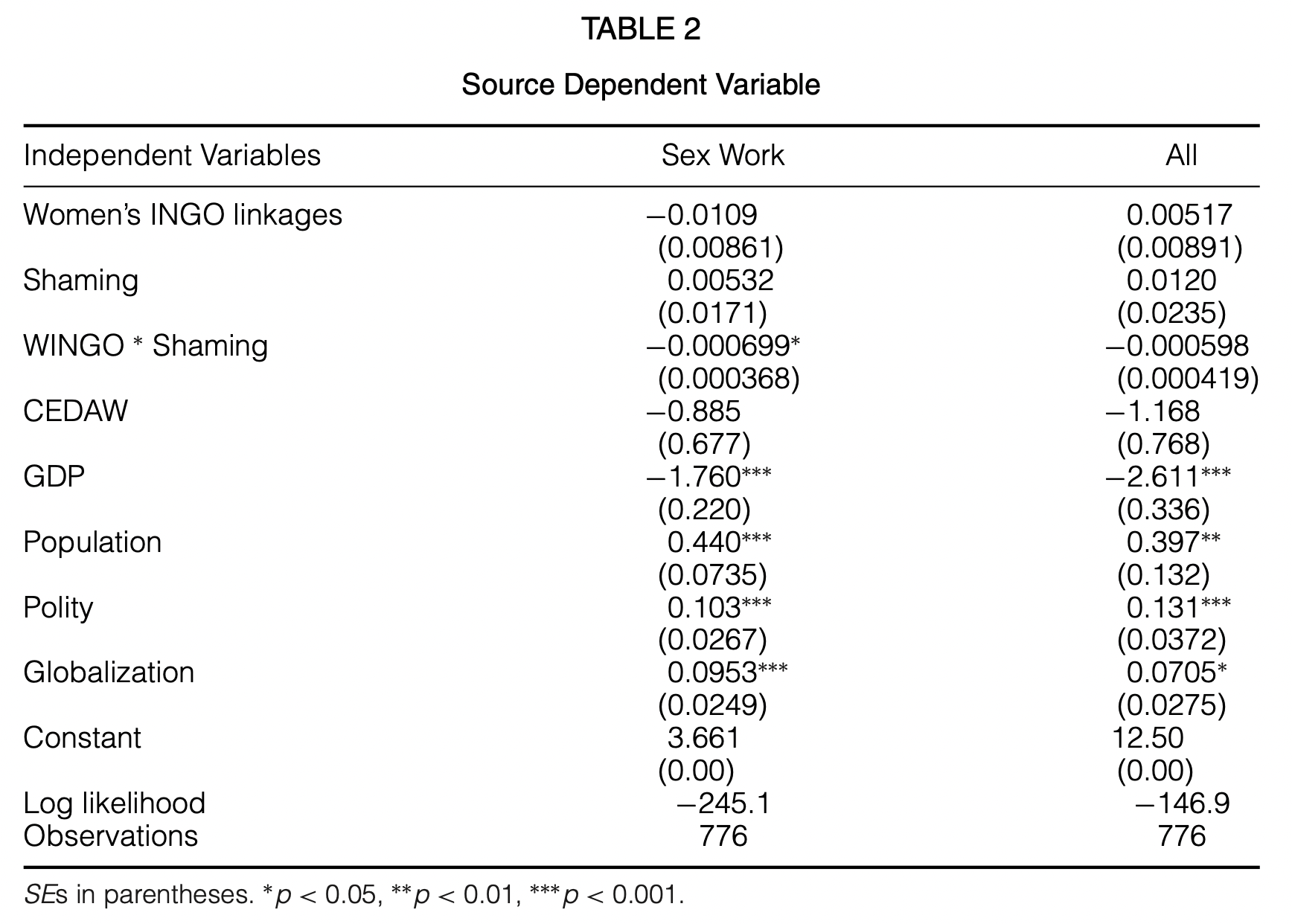Figure 1: Original table 2 from Bell and Banks (2018)

### Original Stata code

use "Final.9.26.2016.dta", clear

xtset ccode year

xtprobit f_psource_di wingos whrnoposmgoldtotal wingos_shaming cedaw_rat_1 log_gdp log_pop polity2 overallglobalizationindex, re
xtprobit f_source wingos whrnoposmgoldtotal wingos_shaming cedaw_rat_1  log_gdp log_pop  polity2 overallglobalizationindex, re

### Replicated models

Using lme4::glmer() works, but there are all sorts of warnings about unidentifiability because of scaling issues, as well as convergence issues.

table2_1 <- glmer(f_psource_di ~ wingos + whrnoposmgoldtotal +
(wingos * whrnoposmgoldtotal) +
cedaw_rat_1 + log_gdp + log_pop + polity2 +
overallglobalizationindex + (1 | ccode),
data = ssq,
family = binomial(link = "probit"))

table2_2 <- glmer(f_source ~ wingos + whrnoposmgoldtotal +
(wingos * whrnoposmgoldtotal) +
cedaw_rat_1 + log_gdp + log_pop + polity2 +
overallglobalizationindex + (1 | ccode),
data = ssq,
family = binomial(link = "probit"))
modelsummary(list("Sex Work" = table2_1, "All" = table2_2),
coef_map = coef_mapping, gof_map = gof_mapping_probit, fmt = 4,
stars = TRUE, notes = c("SEs in parentheses"))
Sex Work All
Women’s INGO linkages 0.0023 0.0062
(0.0117) (0.0119)
Shaming −0.0022 0.0146
(0.0213) (0.0255)
Women’s INGO linkages × Shaming −0.0005 −0.0007
(0.0004) (0.0005)
CEDAW −1.8598 −1.2812
(1.4889) (1.9523)
GDP −2.7069*** −2.9043***
(0.4406) (0.6369)
Population 0.3029 0.5444*
(0.2195) (0.2708)
Polity 0.1177* 0.2029**
(0.0485) (0.0627)
Globalization 0.1370*** 0.0650
(0.0348) (0.0436)
Constant 11.0696* 13.2870*
(4.4853) (5.4300)
Log likelihood −249.497 −146.283
Observations 776 776
+ p < 0.1, * p < 0.05, ** p < 0.01, *** p < 0.001
SEs in parentheses

### Replicated marginal effects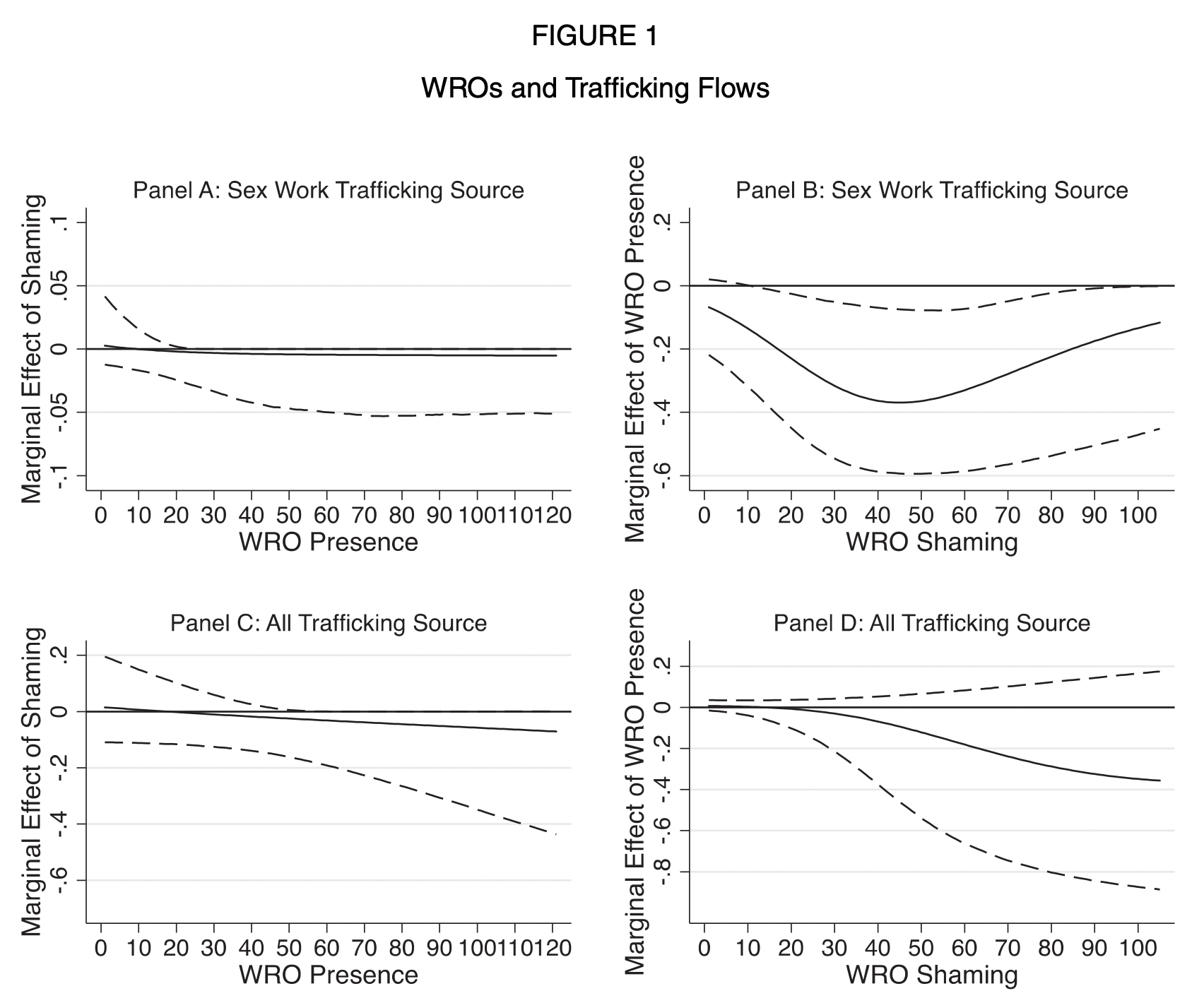Figure 2: Original figure 1 from Bell and Banks (2018)

ame_a <- table2_1 %>%
emtrends(~ wingos,
var = "whrnoposmgoldtotal",
at = list(wingos = seq(0, 107, 1)))

plot_ame_a <- ame_a %>%
as_tibble() %>%
ggplot(aes(x = wingos)) +
geom_hline(yintercept = 0, size = 0.5, color = "#FF4136") +
geom_ribbon(aes(ymin = asymp.LCL, ymax = asymp.UCL),
alpha = 0.05) +
geom_line(aes(y = whrnoposmgoldtotal.trend)) +
geom_line(aes(y = asymp.LCL), linetype = "99") +
geom_line(aes(y = asymp.UCL), linetype = "99") +
labs(x = "Women's INGO presence", y = "Marginal effect of shaming",
title = "Panel A: Sex Work Trafficking Source") +
coord_cartesian(ylim = c(-0.1, 0.1)) +
theme_bw() +
theme(panel.grid.major.x = element_blank(),
panel.grid.minor.x = element_blank(),
panel.grid.minor.y = element_blank())

ame_b <- table2_1 %>%
emtrends(~ whrnoposmgoldtotal,
var = "wingos",
at = list(whrnoposmgoldtotal = seq(0, 107, 1)))

plot_ame_b <- ame_b %>%
as_tibble() %>%
ggplot(aes(x = whrnoposmgoldtotal)) +
geom_hline(yintercept = 0, size = 0.5, color = "#FF4136") +
geom_ribbon(aes(ymin = asymp.LCL, ymax = asymp.UCL),
alpha = 0.05) +
geom_line(aes(y = wingos.trend)) +
geom_line(aes(y = asymp.LCL), linetype = "99") +
geom_line(aes(y = asymp.UCL), linetype = "99") +
labs(x = "Women's INGO shaming", y = "Marginal effect of INGO presence",
title = "Panel B: Sex Work Trafficking Source") +
coord_cartesian(ylim = c(-0.15, 0.1)) +
theme_bw() +
theme(panel.grid.major.x = element_blank(),
panel.grid.minor.x = element_blank(),
panel.grid.minor.y = element_blank())

ame_c <- table2_2 %>%
emtrends(~ wingos,
var = "whrnoposmgoldtotal",
at = list(wingos = seq(0, 107, 1)))

plot_ame_c <- ame_c %>%
as_tibble() %>%
ggplot(aes(x = wingos)) +
geom_hline(yintercept = 0, size = 0.5, color = "#FF4136") +
geom_ribbon(aes(ymin = asymp.LCL, ymax = asymp.UCL),
alpha = 0.05) +
geom_line(aes(y = whrnoposmgoldtotal.trend)) +
geom_line(aes(y = asymp.LCL), linetype = "99") +
geom_line(aes(y = asymp.UCL), linetype = "99") +
labs(x = "Women's INGO presence", y = "Marginal effect of shaming",
title = "Panel C: All Trafficking Source") +
coord_cartesian(ylim = c(-0.15, 0.1)) +
theme_bw() +
theme(panel.grid.major.x = element_blank(),
panel.grid.minor.x = element_blank(),
panel.grid.minor.y = element_blank())

ame_d <- table2_2 %>%
emtrends(~ whrnoposmgoldtotal,
var = "wingos",
at = list(whrnoposmgoldtotal = seq(0, 107, 1)))

plot_ame_d <- ame_d %>%
as_tibble() %>%
ggplot(aes(x = whrnoposmgoldtotal)) +
geom_hline(yintercept = 0, size = 0.5, color = "#FF4136") +
geom_ribbon(aes(ymin = asymp.LCL, ymax = asymp.UCL),
alpha = 0.05) +
geom_line(aes(y = wingos.trend)) +
geom_line(aes(y = asymp.LCL), linetype = "99") +
geom_line(aes(y = asymp.UCL), linetype = "99") +
labs(x = "Women's INGO shaming", y = "Marginal effect of INGO presence",
title = "Panel D: All Trafficking Source") +
coord_cartesian(ylim = c(-0.2, 0.1)) +
theme_bw() +
theme(panel.grid.major.x = element_blank(),
panel.grid.minor.x = element_blank(),
panel.grid.minor.y = element_blank())

(plot_ame_a | plot_ame_b) / (plot_ame_c | plot_ame_d)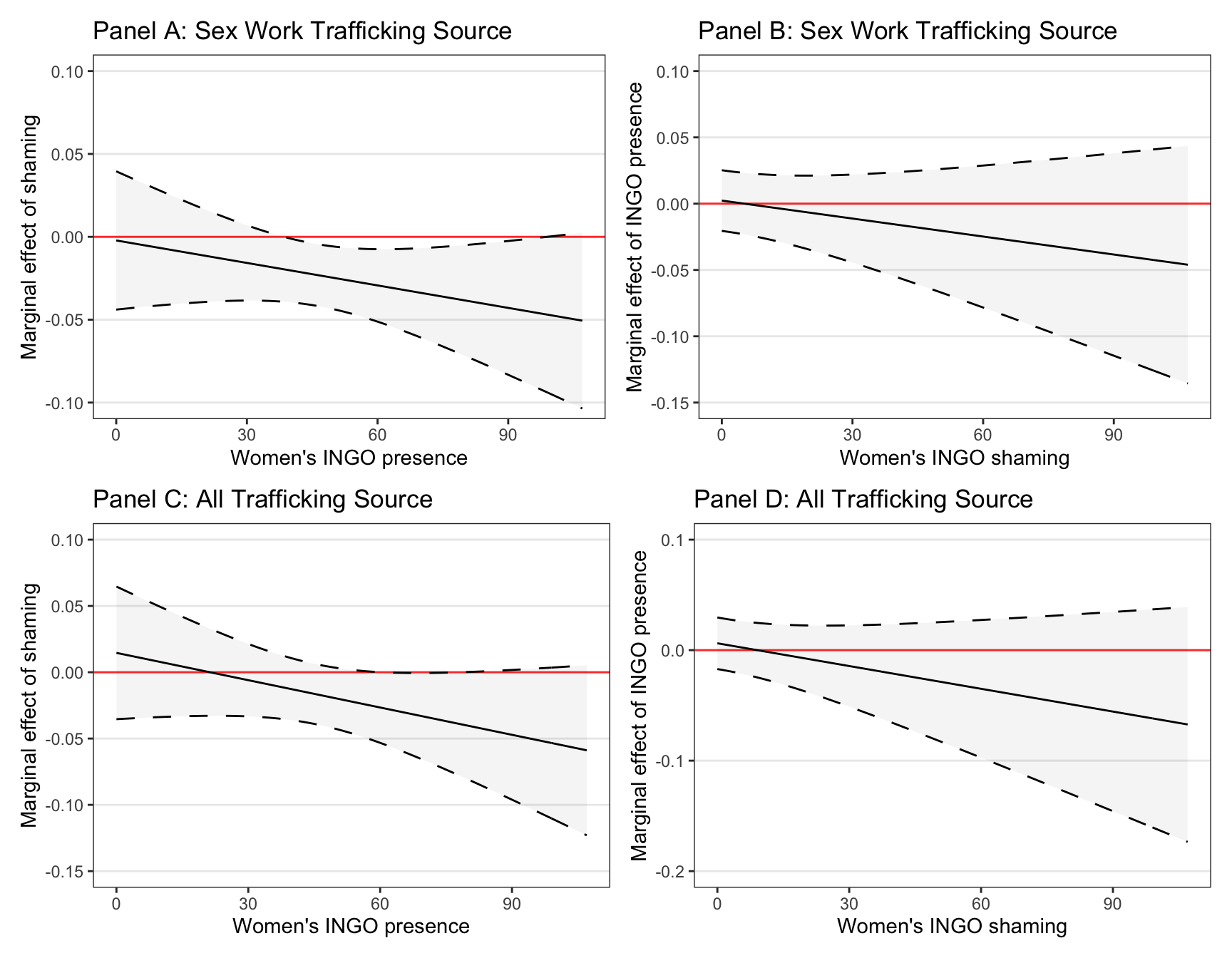Figure 3: Replicated figure 1. emtrends() apparently struggles with glmer() so it’s not picking up on the nonlinear changes in AMEs?

## Table 3

Effect of women’s INGO linkages, human rights shaming, and their interaction on the a country’s 3P trafficking index (which ranges from 1–15; higher values = better prosecution, prevention, and protection)

### Original results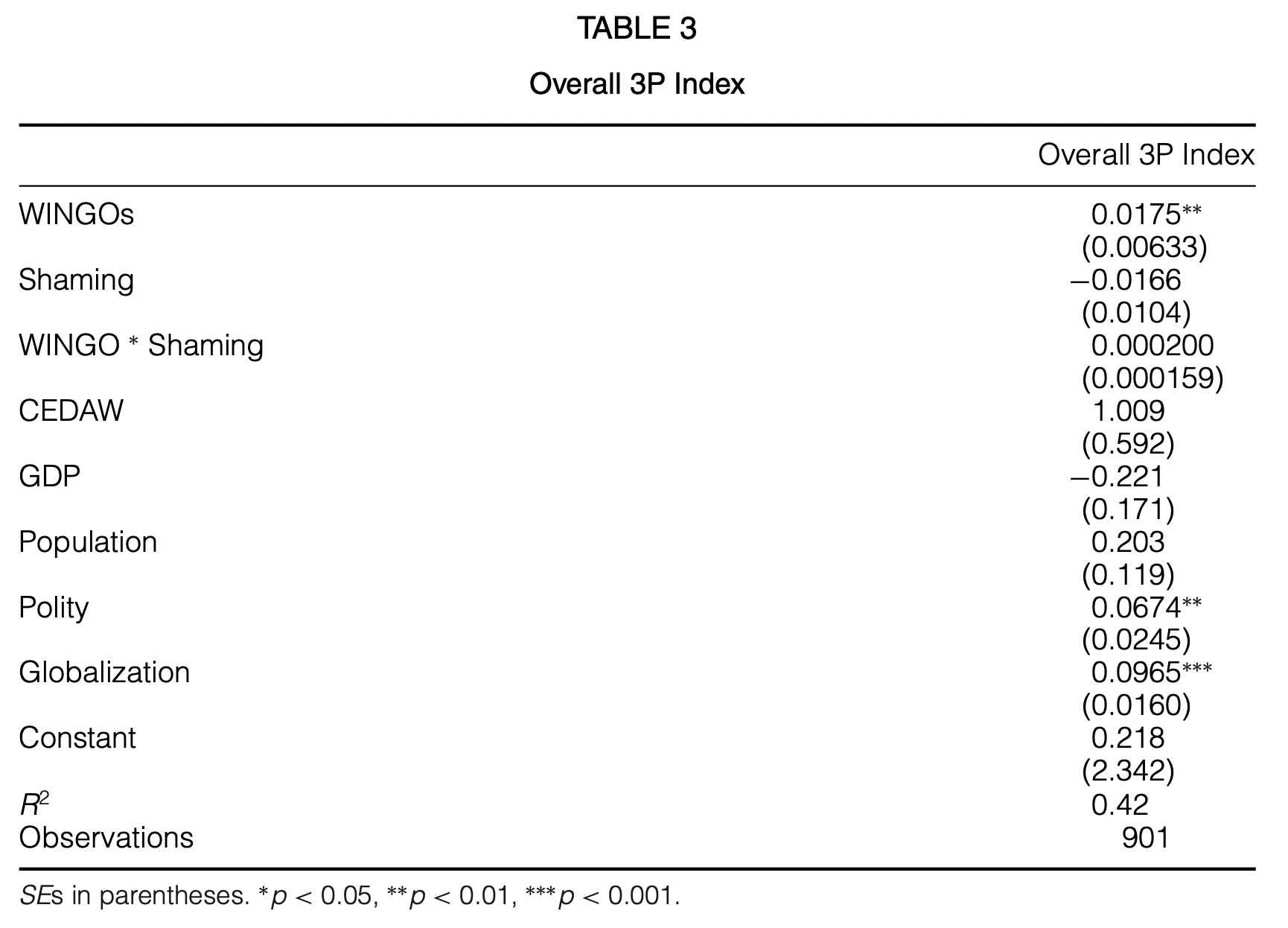Figure 4: Original table 3 from Bell and Banks (2018)

### Original Stata code

use "Final.9.26.2016.dta", clear

xtset ccode year

xtreg f_Overall3P wingos whrnoposmgoldtotal wingos_shaming cedaw_rat_1 log_gdp log_pop polity2 overallglobalizationindex, re

### Replicated model

Using lme4::glmer() works and only gives a warning about scaling issues; the model converges.

table3 <- lmer(f_Overall3P ~ wingos + whrnoposmgoldtotal +
(wingos * whrnoposmgoldtotal) +
cedaw_rat_1 + log_gdp + log_pop + polity2 +
overallglobalizationindex + (1 | ccode),
REML = FALSE,
data = ssq)
modelsummary(list("Overall 3P Index" = table3),
coef_map = coef_mapping, gof_map = gof_mapping_ols, fmt = 4,
notes = c("SEs in parentheses",
"No stars here because <code>lmer()</code> doesn't output p-values",
"No R² here because <code>lmer()</code> doesn't use it (and Stata fakes it)"))
Overall 3P Index
Women’s INGO linkages 0.0176
(0.0062)
Shaming −0.0176
(0.0103)
Women’s INGO linkages × Shaming 0.0002
(0.0002)
CEDAW 1.0144
(0.5793)
GDP −0.2018
(0.1641)
Population 0.1965
(0.1140)
Polity 0.0711
(0.0237)
Globalization 0.0919
(0.0155)
Constant 0.4125
(2.2411)
Observations 901
SEs in parentheses
No stars here because lmer() doesn’t output p-values
No R² here because lmer() doesn’t use it (and Stata fakes it)

### Replicated marginal effects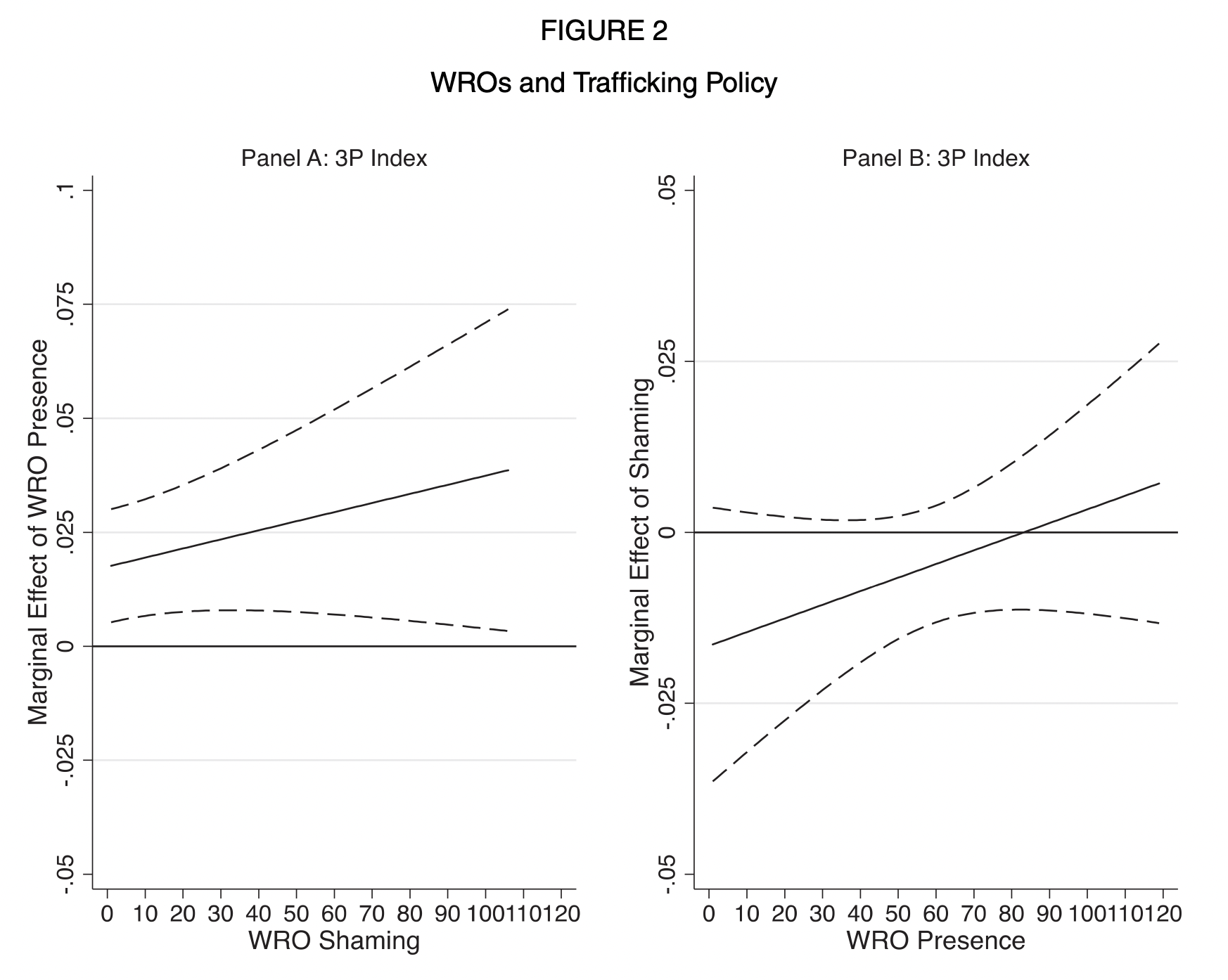Figure 5: Original figure 2 from Bell and Banks (2018)

ame_tbl3_a <- table3 %>%
emtrends(~ whrnoposmgoldtotal,
var = "wingos",
at = list(whrnoposmgoldtotal = seq(0, 107, 1)))

plot_ame_tbl3_a <- ame_tbl3_a %>%
as_tibble() %>%
ggplot(aes(x = whrnoposmgoldtotal)) +
geom_hline(yintercept = 0, size = 0.5, color = "#FF4136") +
geom_ribbon(aes(ymin = lower.CL, ymax = upper.CL),
alpha = 0.05) +
geom_line(aes(y = wingos.trend)) +
geom_line(aes(y = lower.CL), linetype = "99") +
geom_line(aes(y = upper.CL), linetype = "99") +
labs(x = "Women's INGO shaming", y = "Marginal effect of INGO presence",
title = "Panel A: 3P index") +
coord_cartesian(ylim = c(-0.05, 0.1)) +
theme_bw() +
theme(panel.grid.major.x = element_blank(),
panel.grid.minor.x = element_blank(),
panel.grid.minor.y = element_blank())

ame_tbl3_b <- table3 %>%
emtrends(~ wingos,
var = "whrnoposmgoldtotal",
at = list(wingos = seq(0, 107, 1)))

plot_ame_tbl3_b <- ame_tbl3_b %>%
as_tibble() %>%
ggplot(aes(x = wingos)) +
geom_hline(yintercept = 0, size = 0.5, color = "#FF4136") +
geom_ribbon(aes(ymin = lower.CL, ymax = upper.CL),
alpha = 0.05) +
geom_line(aes(y = whrnoposmgoldtotal.trend)) +
geom_line(aes(y = lower.CL), linetype = "99") +
geom_line(aes(y = upper.CL), linetype = "99") +
labs(x = "Women's INGO presence", y = "Marginal effect of shaming",
title = "Panel B: 3P index") +
coord_cartesian(ylim = c(-0.05, 0.05)) +
theme_bw() +
theme(panel.grid.major.x = element_blank(),
panel.grid.minor.x = element_blank(),
panel.grid.minor.y = element_blank())

plot_ame_tbl3_a | plot_ame_tbl3_b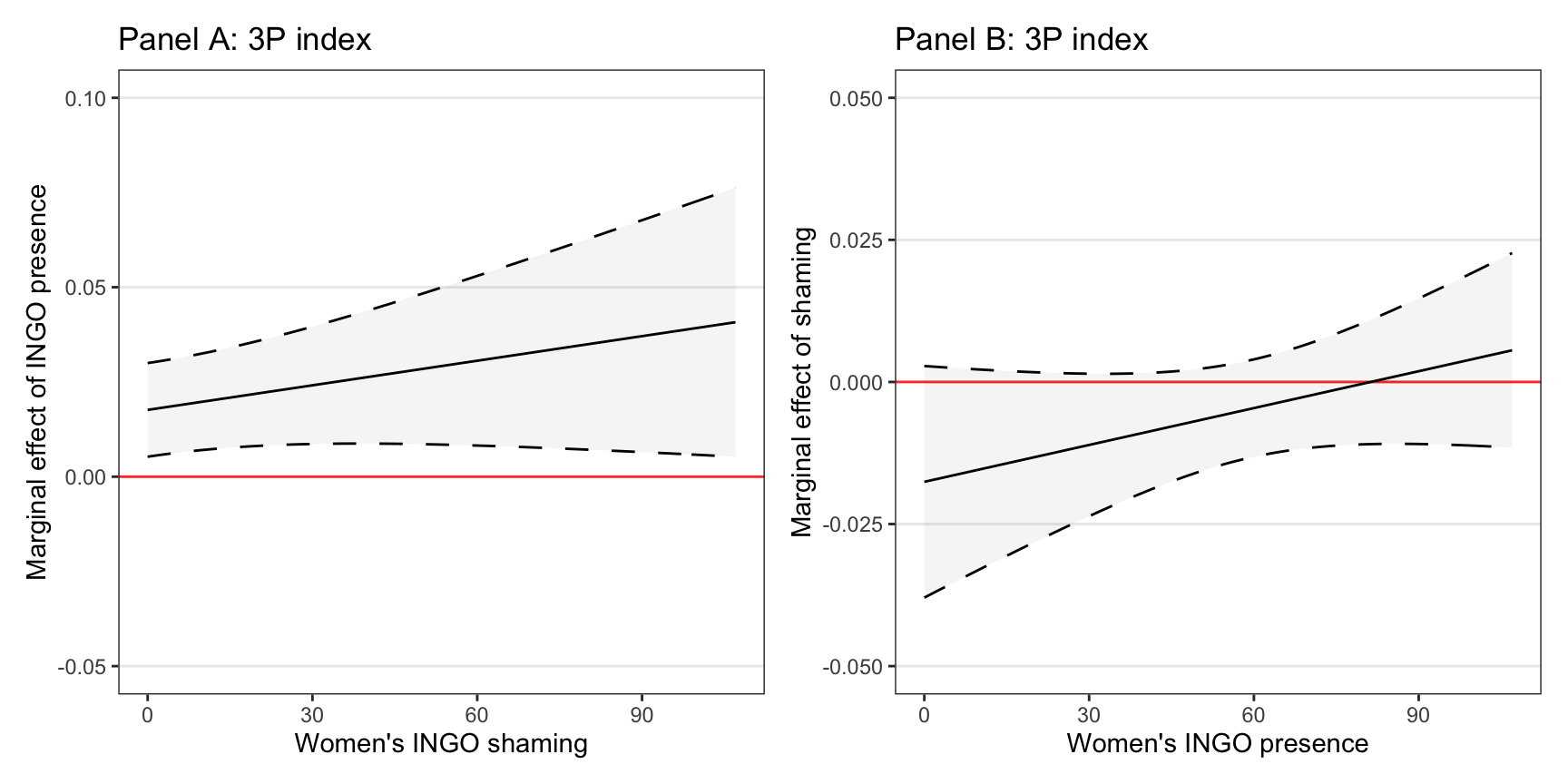Figure 6: Replicated figure 2. This is pretty close!